# NEET Important Chapter - Laws of Motion

Get plus subscription and access unlimited live and recorded courses## Laws of Motion: Important Concepts and Formulae

Laws of Motion Notes is the most useful and important tool for the students preparing for the NEET exam. In the chapter laws of motion for the NEET exam, we will cover newton’s laws, friction forces, inertial, the concept of impulse, conservation laws, circular motion, etc.

The three statements made by Isaac Newton that serve as the foundation of classical mechanics and describe the link between forces acting on a body and its motion are known as Newton's laws of motion. This unit will have at least one question for candidates. Laws of Motion, Friction, and Circular Motion Dynamics are just a few of the key concepts covered in this section.

In this article, we have provided important concepts regarding the laws of motion chapter that will be asked in the NEET 2022 exam.

### Important Topics of Laws of Motion

• Newton’s second law of motion

• Newton’s Third law of motion

• Friction and its types

• Linear momentum

• Impulse physics

• Conservation of linear momentum

• Laws of Friction

• Circular motion

• Centripetal and centrifugal forces

### Important Concepts of Laws of Motion

 Name of the Concept Key Points of Concept Newton Laws of Motion Newton's first law gives us a relation between inertia and force. In our day to day life we experience Newton's first law and Newton's first law of motion examples.Newton's second law of motion states that force acting on an object is directly proportional to rate of change of linear momentum.$\vec {F}\propto \dfrac {d \vec P}{dt}$Newton 3 law of motion states that every action is having equal and opposite reaction.Newton's third law formula is given by:$\vec {F_{12}}=-\vec {F_{21}}$ Conservation of Linear Momentum The law of conservation of linear momentum states that “when external forces are not acting, then total momentum of the body remains constant.” In other words, the momentum of the body before impact will be the same as the after impact. Mathematically we write:${m_1}{v_1}={m_2}{v_2}\Rightarrow {v_1}=\dfrac {{m_2}{v_2}}{m_1}$ Concurrent Forces and Equilibrium When a group of forces will be acting on a single point, such forces are known as the concurrent forcesIf the resultant force of these forces is zero, then these concurrent forces are said to be in “equilibrium”. Friction Friction is the opposition offered when any object is moving a plane.The formula of the friction force is:$\vec {F}=\mu N$Relation between the coefficient of friction and of friction $\theta$:$\theta=\tan^{-1}\mu$ Types of friction Static Friction:The force that acts between the surfaces when they are at rest with respect to each other.Kinetic Friction or Sliding Friction: the resistance that is created between any two objects when they are sliding against each other.Rolling Friction: The force which resists the motion of a ball or wheel and is the weakest types of friction. Laws of Friction The total magnitude of limiting frictional force is always proportional to the normal force acting at the contact surface.The magnitude of limiting frictional force is always independent of the total surface area of contact between the surfaces. Dynamics of uniform circular motion Circular Motion: An object moving along the circumference of the circle with uniform velocity is said to be under uniform circular motion.Centripetal Force: It is a pseudo force. It acts along a radius and is directed towards the centre of the circle.Centrifugal Force: It is a friction force acting on the body under rotation, it acts along the radius and away from the centre of the circle.

### List of Important Formulae

 Sl. No Name of the Concept Formula 1 Newton’s second law of motion $\vec {F}=k \dfrac {d \vec{P}}{dt}$ 2 Resultant force $\displaystyle\sum\limits_{i=1}^k \vec {F}= \vec {F_1}+\vec {F_2}+....+\vec {F_k}$For equilibrium, $\displaystyle\sum\limits_{i=1}^k \vec {F_i}= 0$ 3 Newton’s third law of motion $F_{12}=-F_{21}$ 4 Momentum and Impulse $\vec {P}= m v$$I=\int_{t_1}^{t_2}\vec {F} dt$ 5 Law of conservation of momentum m1v1=m2v2 6 Miscellaneous formulae Bullets travelling with a velocity v hitting the wall:${F_{wall}}=n m v$n- Number of bulletsLiquid jet of area A moving with velocity v hits the wall:${F_{wall}}= 2 A {v^2} \cos \theta$ 7 Friction ${F_r}=\mu N$Kinetic friction ${F_r}={\mu_{k}} N$Static friction ${F_s}=\mu_s N$ 8 Centripetal Force $F= m {\omega^2}r$ 9 Banking of road Velocity of Vehicle on banked Road: $v=\sqrt {\dfrac{rg\left(tan\theta+\mu_s\right)}{1-\mu_S\,tan\theta}}$Angle of Banking : $\theta=\tan^{-1}\dfrac{v^2}{rg}$

### Solved Examples of Laws of Motion

1. When a body is placed on a rough plane inclined at an angle θ to the horizontal, what will be the acceleration gained by the body.

Sol:

Let us first start with the FBD of the problem.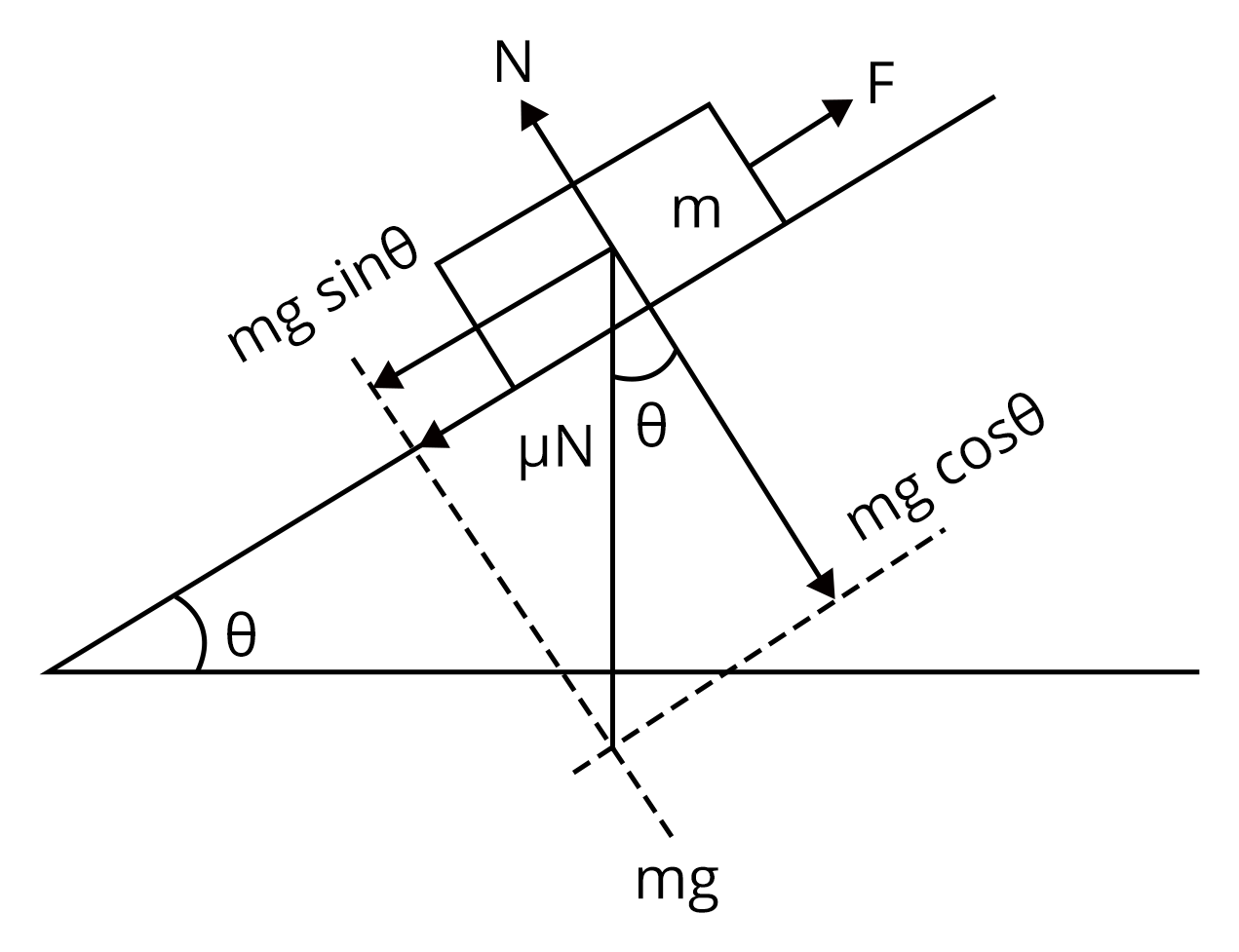Now, we are asked to determine the acceleration of the body. We know that the,

$F_{net}=ma$ and the acceleration a will be along the surface. Thus, the net force we need will be along the surface.

Thus, we get:

$F_{net}=mg \sin \theta -{f_k}$

$ma=mg \sin \theta-\mu N$....(1)

Now, from FBD, value of N (normal force) will be: $N=mg \cos \theta$

Therefore, equation (1) becomes:

$ma=mg \sin \theta-\mu \left(mg \cos \theta \right)$

$a=g\left (\sin \theta-\mu \cos \theta\right)$

Therefore, acceleration of the given body on inclined plane is $g\left (\sin \theta-\mu \cos \theta\right)$.

Key Point: Since the body is acting on the rough surface the frictional force acting will be kinetic friction or sliding friction.

2. One end of a string of length l is connected to a particle of mass m and the other to a small peg on a smooth horizontal table. If the particle moves in a circle with speed v the net force on the particle?

Sol:

Given,

The length of a the string=l

The mass of the particle is m and it is moving with velocity v in a circular path. Now we are asked to determine the net force acting on the particle.

Since one end of the string is attached to the particle, the net force acting will be tension force, and this will be equal to the centripetal force acting on the particle.

Therefore, we write

Net force=Centripetal force

$T=\dfrac {mv^2}{r}$

Therefore, the net force acting is tension force T.

Key Point: When a particle is attached to one end of the string and it is further subjected to rotate then, the net force acting will be the tension force.

### Previous Year Questions of Laws of Motion

1. A particle moving with velocity $\vec V$ is acted by three forces shown by the vector triangle PQR. The velocity of the particle will : (NEET 2019)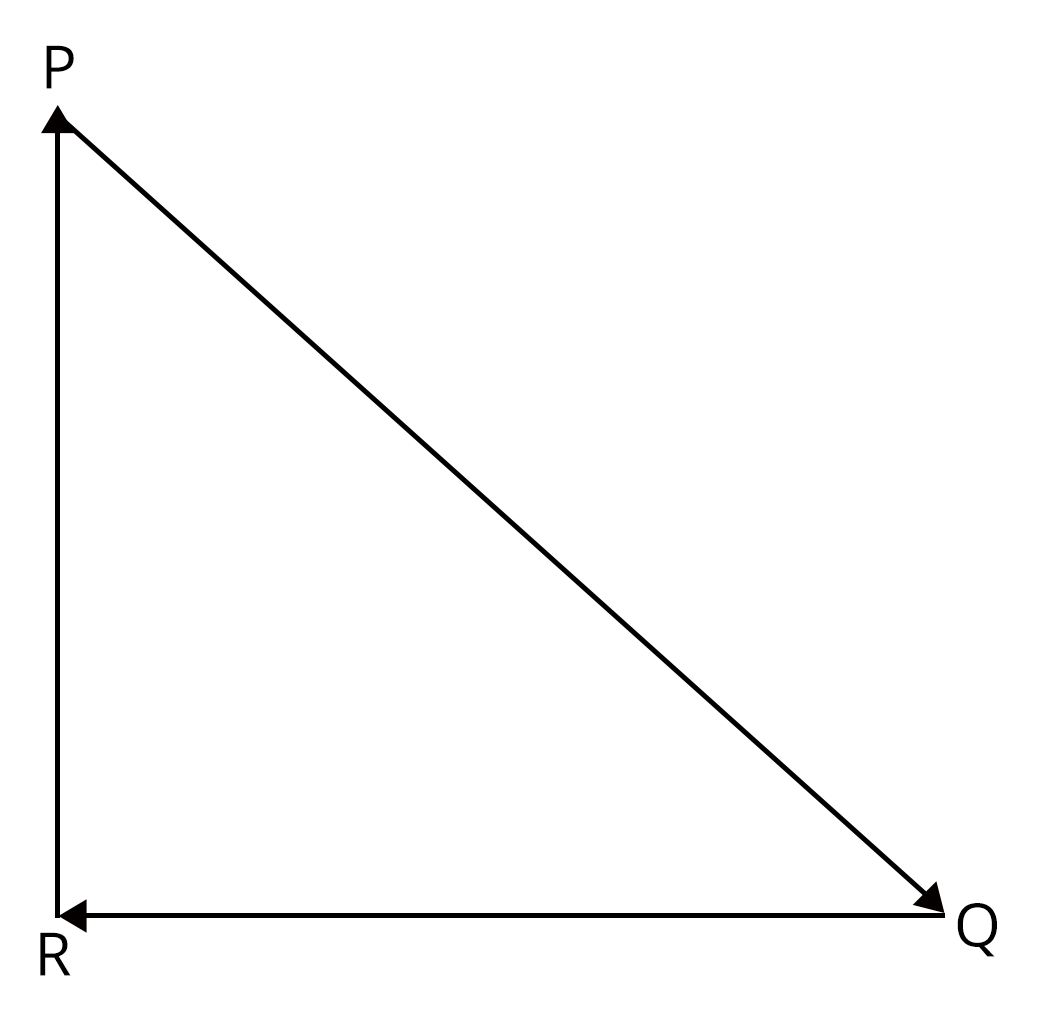1. Increase

2. Decrease

3. Remains constant

4. Change according to the smallest force $\vec {QR}$

Sol:

In this question, we are given that a particle is moving in closed loop, it implies that the net force acting on the particle will be zero.

I.e., $\vec F_{net}=0$

$m \left[ \dfrac {d\vec v}{dt}\right]=0$

$\dfrac {d \vec v}{dt}=0$

v= constant

Therefore, option C is right answer.

Trick: In closed paths, the net force acting on the body will be constant and velocity will constant.

2. A block of mass m is placed on a smooth inclined wedge ABC of inclination θ as shown in the figure. The wedge is given an acceleration 'a' towards the right. The relation between a and θ for the block to remain stationary on the wedge is? (NEET 2018)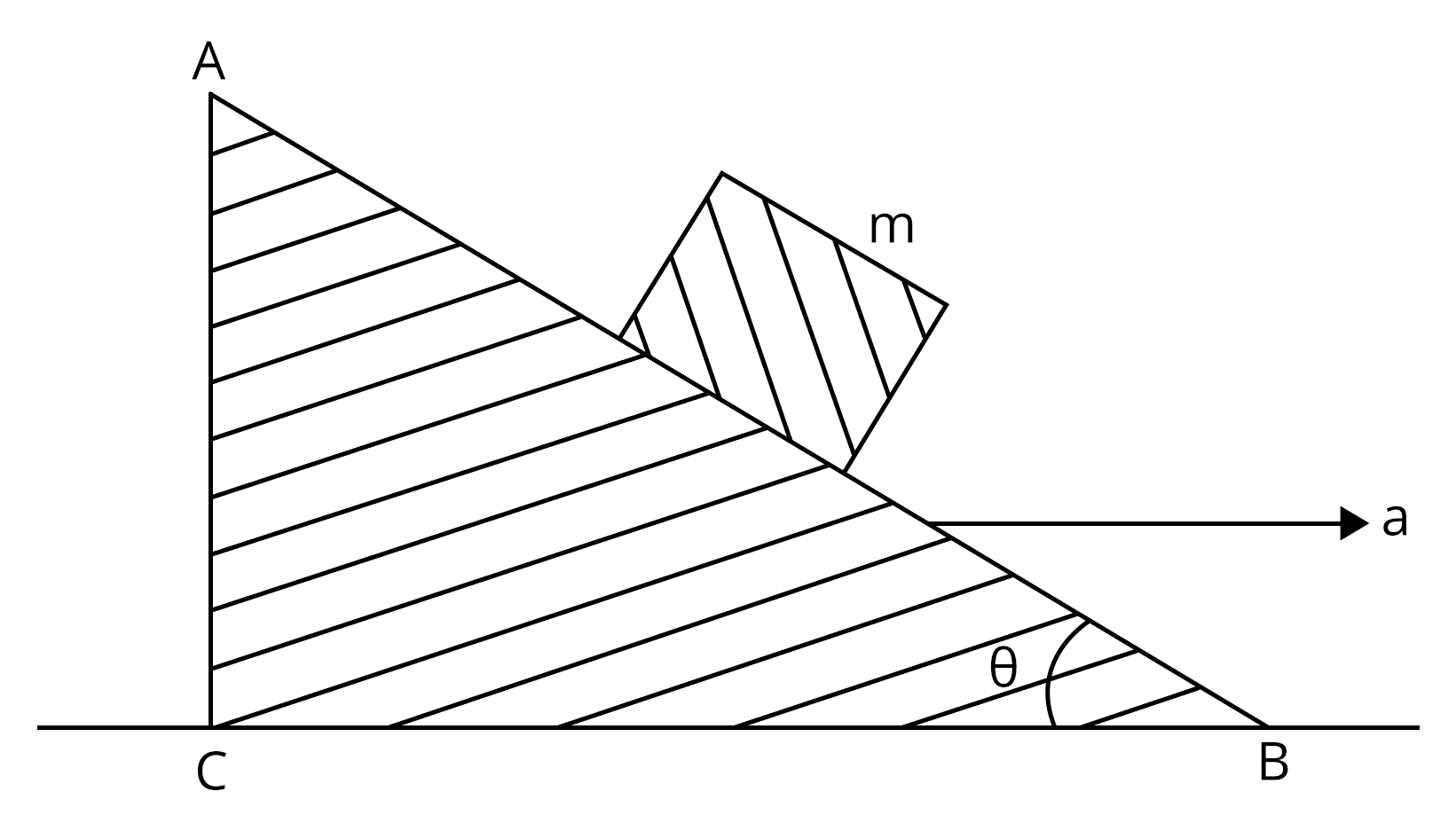1. $a=g \cos \theta$

2. $a=\dfrac {g} {cosec \theta}$

3. $a=g \tan \theta$

4. $a=\dfrac {g} {\sin \theta}$

Sol: Let us start with free body diagram for the given problem.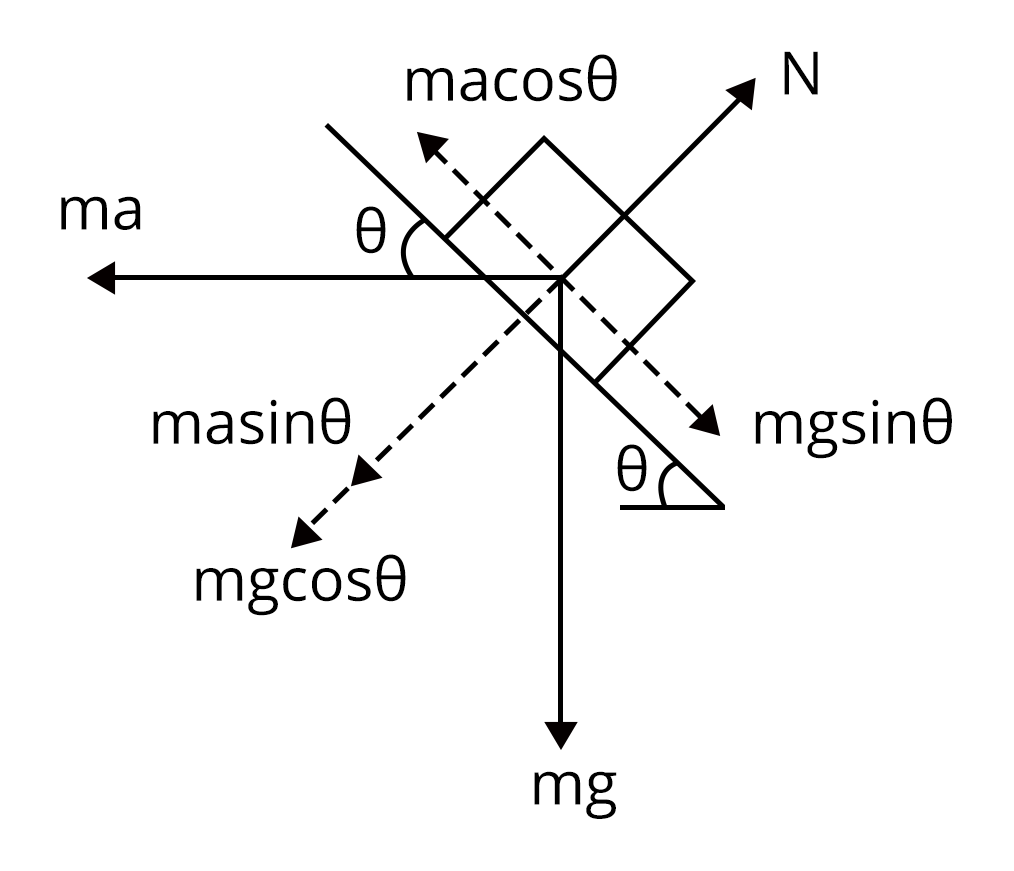Here, we will use the concept of pseudo force to arrive at the solution. In non inertial frame, we will consider the object is at rest and the forces acting on the bock will be:

$ma \cos \theta = mg \sin \theta$

$a=g \dfrac { \sin \theta}{ \cos \theta}=g \tan \theta$

Therefore, option c is the right answer.

Trick: We should use pseudo force in the non-inertial frame so that we can consider the block is at rest.

### Practice Questions

1. A rigid ball of mass m strikes a wall at 600 and gets reflected without loss of speed as shown in the figure. The value of impulse imparted by the wall on the ball will be( Ans: mv)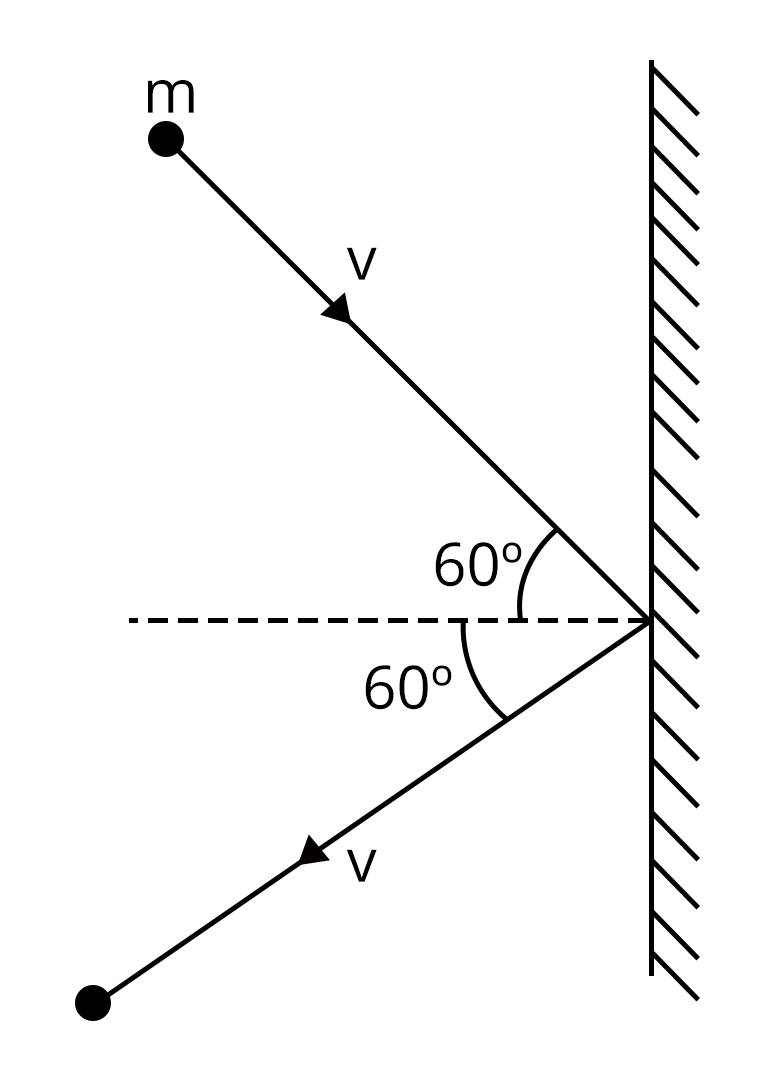2. A block of mass m is placed on a smooth inclined plane of inclination θ and of mass M, which in turn is placed on a smooth horizontal surface AB as shown. A horizontal force F is applied on the side of the inclined plane so that the block m is just prevented from slipping over the plane. The value of F is (Ans:  $\left (M+m\right) g \tan \theta$)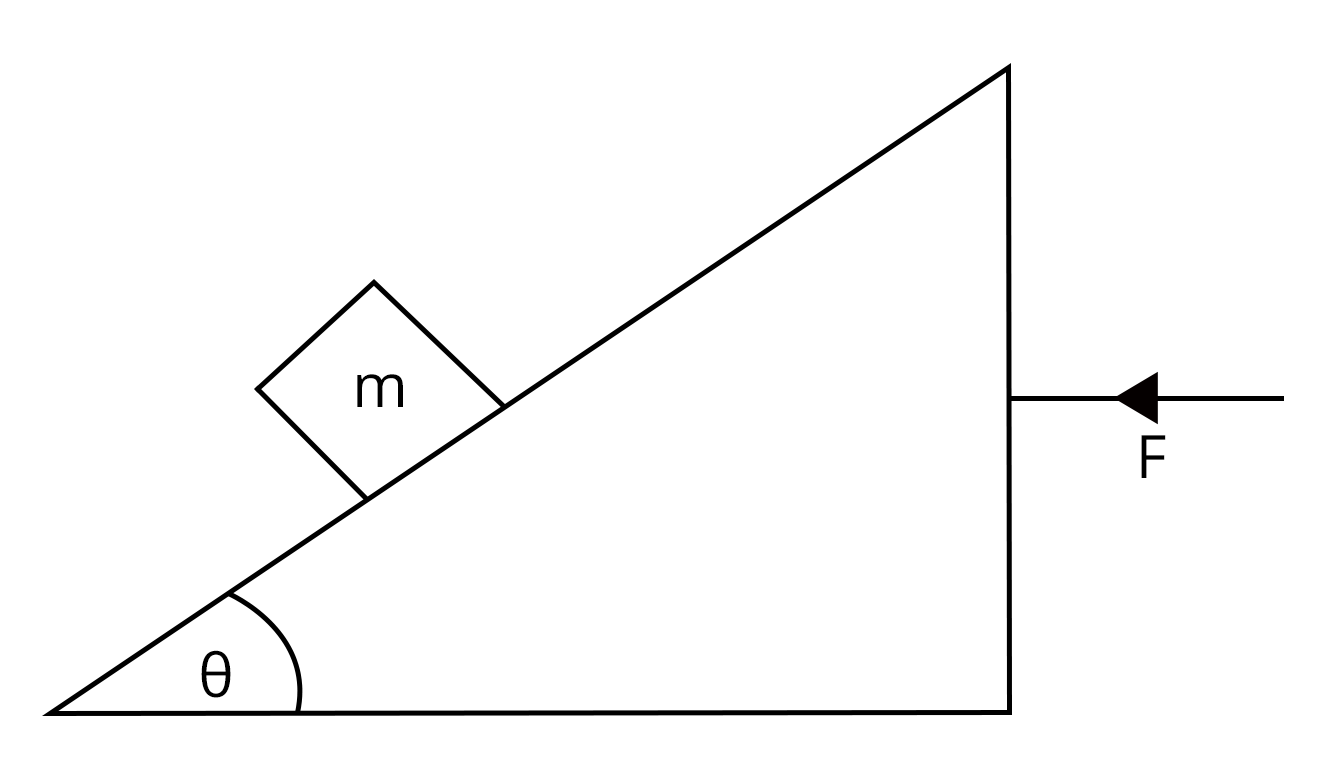### Conclusion

In this article, we discussed important concepts of the NEET exam from the chapter laws of motion. We have also solved a few important questions, recently asked questions and solved them with tricks. In this article, we have provided the practice questions that will help students to check their level of preparation.

See More## NEET Important Dates

View All Dates
NEET 2022 exam date and revised schedule have been announced by the NTA. NEET 2022 will now be conducted on 17-July-2022, and the exam registration closes on 20-May-2022. You can check the complete schedule on our site. Furthermore, you can check NEET 2022 dates for application, admit card, exam, answer key, result, counselling, etc along with other relevant information.
See More
View All Dates## NEET Information

Application Form
Eligibility Criteria
Reservation Policy
NTA has announced the NEET 2022 application form release date on the official website https://neet.nta.nic.in/. NEET 2022 Application Form is available on the official website for online registration. Besides NEET 2022 application form release date, learn about the application process, steps to fill the form, how to submit, exam date sheet etc online. Check our website for more details.## NEET 2022 Study Material

View NEET Syllabus in Detail
View NEET Syllabus in Detail## NEET 2022 Study Material

View all study material for NEET
All
Physics
Chemistry
Biology
See All## NEET Question Papers## NEET 2022 Book Solutions and PDF Download

View all NEET Important Books
Biology
NCERT Book for Class 12 Biology
Physics
NCERT Book for Class 12 Physics
Chemistry
NCERT Book for Class 12 Chemistry
Physics
H. C. Verma Solutions
See All## NEET Mock Tests

View all mock tests
"NEET 2022 free online mock test series for exam preparation are available on the Vedantu website for free download. Practising these mock test papers of Physics, Chemistry and Biology prepared by expert teachers at Vedantu will help you to boost your confidence to face the NEET 2022 examination without any worries. The NEET test series for Physics, Chemistry and Biology that is based on the latest syllabus of NEET and also the Previous Year Question Papers."
See More## NEET 2022 Cut Off

NEET Cut Off
NTA is responsible for the release of the NEET 2022 cut off score. The qualifying percentile score might remain the same for different categories. According to the latest trends, the expected cut off mark for NEET 2022 is 50% for general category candidates, 45% for physically challenged candidates, and 40% for candidates from reserved categories. For the general category, NEET qualifying marks for 2021 ranged from 720-138 general category, while for OBC/SC/ST categories, they ranged from 137-108 for OBC, 137-108 for SC and 137-108 for ST category.
See More## NEET 2022 Results

The NEET 2022 result is published by NTA on https://neet.nta.nic.in/ in the form of a scorecard on 7-Sep-2022. The scorecard will include the roll number, application number, candidate's personal details, and the percentile, marks, and rank of the candidate. Only those candidates who achieve the NEET cut-off will be considered qualified for the exam.
See More
Rank List
Counselling
Cutoff
NEET 2022 state rank lists will be released by the state counselling committees for admissions to the 85% state quota and to all seats in private medical and dental colleges. NEET 2022 state rank lists are based on the marks obtained in entrance exams. Candidates can check the NEET 2022 state rank list on the official website or on our site.## NEET Top Colleges

View all NEET 2022 Top Colleges
Want to know which Engineering colleges in India accept the NEET 2022 scores for admission to Engineering? Find the list of Engineering colleges accepting NEET scores in India, compiled by Vedantu. There are 1622 Colleges that are accepting NEET. Also find more details on Fees, Ranking, Admission, and Placement.
See More## FAQs on NEET Important Chapter - Laws of Motion

FAQ

1. Is laws of motion important for NEET?

With a weightage of 3%, Laws of Motion is an important chapter in the NEET Syllabus. It is critical to have a firm grasp on the principles of this topic, not only to pass the exam, but also to comprehend its numerous applications in science.

2. How many questions come in NEET from laws of motion?

Laws of motion is one of the most significant chapters in physics, and the Physics NEET exam has a total of 45 questions. This chapter is worth 3% of your grade, and you can easily pass it.

3. Can I study laws of motion without studying kinematics?

Yes. They are, in fact, tied to one another. The motion in the 1D, 2D, and 3D portions is a significant aspect of the application of laws of motion issues. This topic is covered in at least 2-4 NEET questions.## Notice board

News
BlogTrending pages